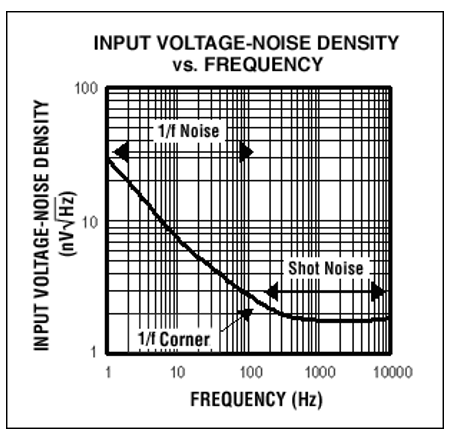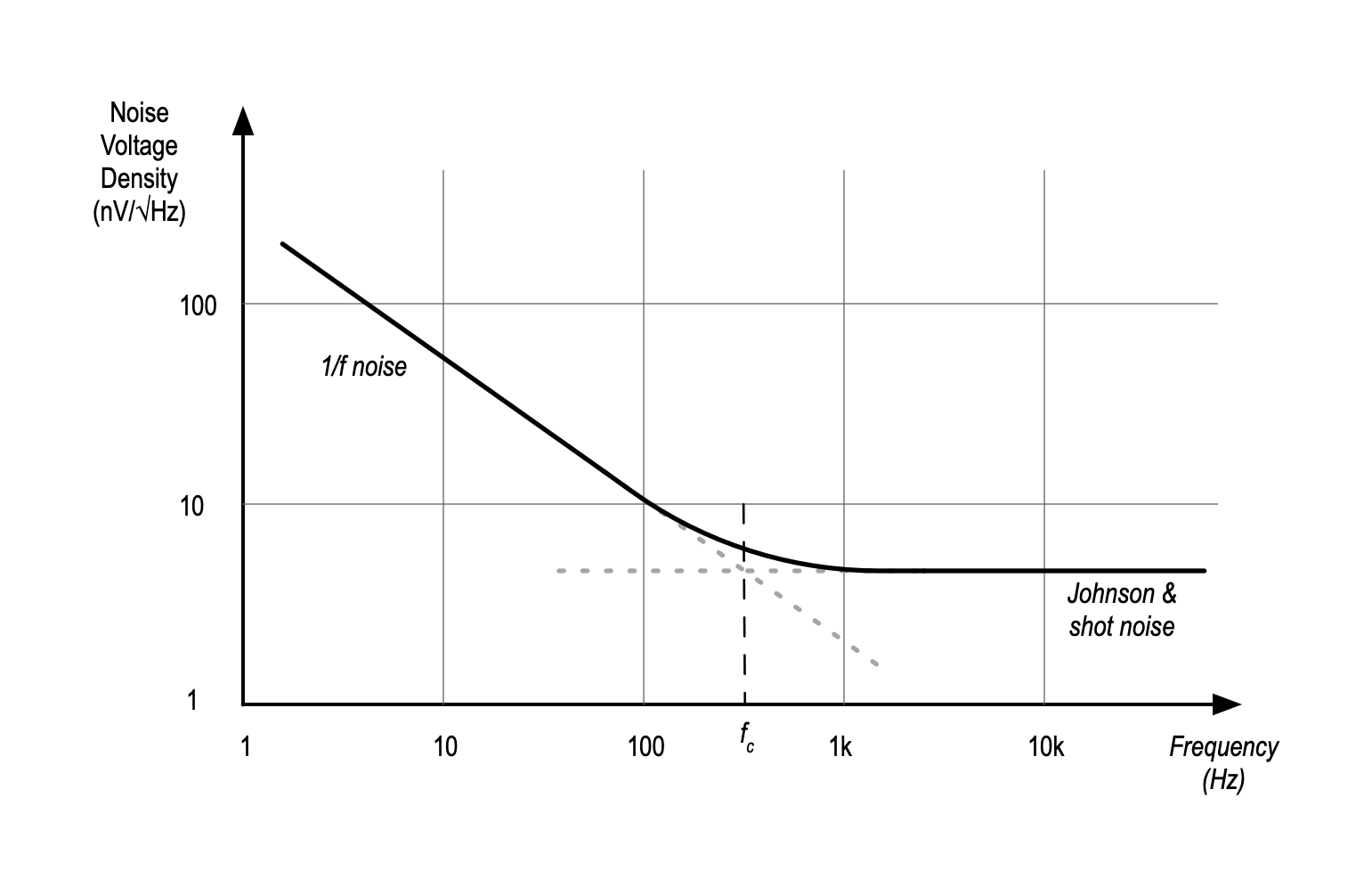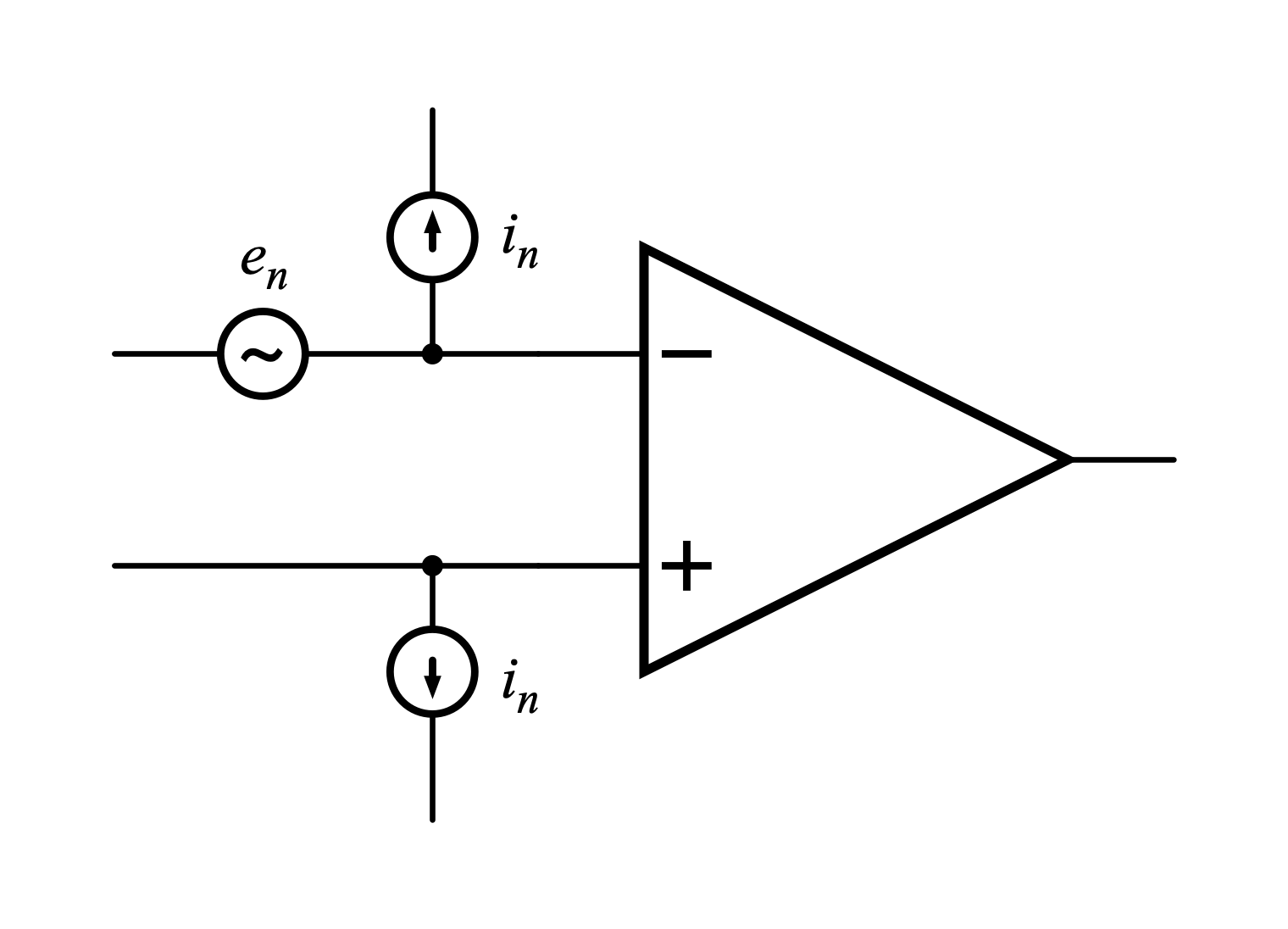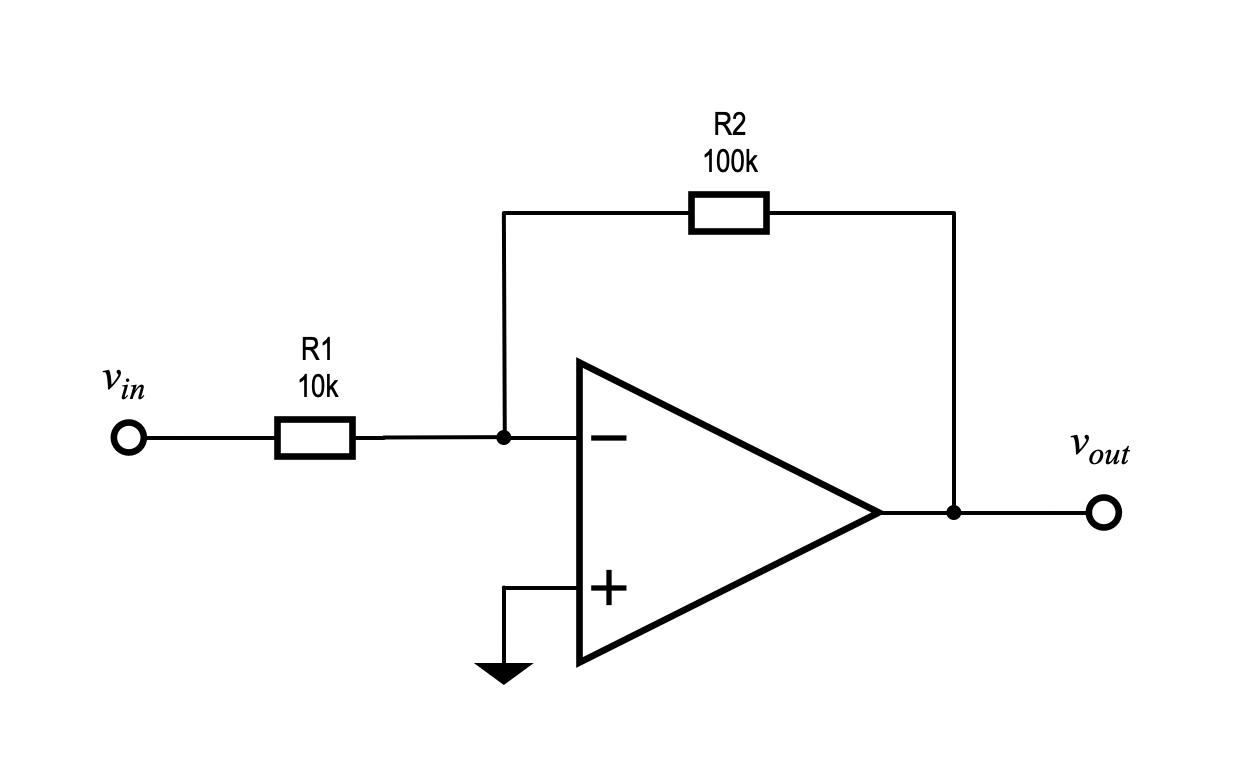# Noise in Op Amp CircuitsWritten by

Noise in passive components is dominated by Johnson or thermal noise and has been discussed in detail in a previous post. When it comes to noise in active circuits like op amps there are a two additional noise sources we need to know about. As well as Johnson noise in the bulk semiconductor material, there is shot noise caused by charges crossing PN junctions and flicker noise associated with impurities in the channel and charge recombination.

Shot noise is the random fluctuation in voltage which results from the discrete nature of charges when they act independently (as they do in a semiconductor junction) It does occur in conductors but at a level so low it can be ignored. Shot noise is related to the amount of charge flowing (current) and is not temperature dependent. Like Johnson noise it has equal energy across the frequency spectrum, known as white noise.

Flicker noise, often called 1/f noise, is an artefact of the materials and construction of the semiconductor devices. It is called 1/f noise because its power density falls off with the inverse of frequency. This type of noise is known as pink noise. For example, for pink noise we would see the same energy in the 10 to 100Hz band as we would see in the 100 to 1000Hz band, but for white noise but we would see 10 times the energy in the latter band since it is 10 times wider.

This means that flicker noise dominates at low frequencies and shot plus Johnson noise dominates at high frequencies. In a device such as an op amp there will be a Johnson and shot noise component that is flat with frequency and a flicker noise component that will fall off at 1/f as shown in Figure 1. At some frequency the amplitudes of these two noise components will cross over. The point where the 1/f noise has the same value as the white noise which is known as the corner frequency and is designated fc.Figure 1The Johnson and shot noise voltage density is flat with frequency and the flicker noise voltage density falls off at 1/f as shown here. At some frequency fc the amplitudes of these two noise components will cross over. If the bandwidth of concern straddles fc, we need to take this into account as shown in the text in calculating the overall noise.

Fortunately, we don’t have to worry about all the different sources in an op amp (and there will be dozens of them) when designing practical op amp circuits. Manufacturers conveniently specify op amp noise in terms of three parameters – a noise voltage density en, a current density in, both referred to the input and a figure or graph for fc.

As shown in Figure 2, the noise model of an op amp looks like a noiseless op amp with a noise voltage source en in series with input and noise current sources in driving current out of the input terminals. The noise currents will interact with the source resistances to create a noise voltage. To calculate the noise in an op amp circuit we need to sum up all of the noise voltage densities at the input and multiply through the circuit gain. Just don’t forget to add the noise voltage densities produced by Johnson noise in the source resistance itself. Let’s look at a real example to see how this is done.Figure 2The noise model of an op amp looks like a noiseless op amp with a noise voltage density source en in series with input and noise current density sources in driving current out of the input terminals. The noise currents will interact with the source resistances to create a noise voltage density that must be added to en, and the Johnson noise produced in the source resistances. If there is a preceding stage, you also need to add the noise it contributes.Figure 3A simple inverting amplifier circuit with a gain of -10 serves as an example for the calculation of noise voltages in an op amp circuit. You first calculate and sum all the noise voltages at the input of the op amp then multiply through the circuit gain to find the output noise. If necessary, you can add the noise coupled in via the op amp power supplies.

Figure 3 shows a simple inverting amplifier circuit with a gain of -10. In this case I have chosen a TLV2460 general purpose RRIO op amp with en = 11nV/√Hz, in = 0.13pA/√Hz and fc = 150Hz. Let’s assume we are interested in noise over the bandwidth range 10Hz to 10kHz. To calculate the rms noise voltage that we will see at the op amp output we need to follow the steps below. The table shows the calculation for each step and the results

1. Calculate the Johnson noise voltage density in the parallel combination of R1and R2. This is the input resistance “seen” by the inverting input of the op amp.
2. Calculate the voltage noise density created by in flowing in the parallel combination of R1 and R2. Since the non-inverting input is connected directly to ground, no noise voltage is present at this pin.
3. Find (look up in the data sheet) the op amp noise voltage density referred to the op amp input.
4. Sum up the above three voltage densities (using a root sum of squares since the noise voltages are uncorrelated) to get a total noise voltage density at the op amp input.
5. Multiply the input noise density by the gain to get the output noise voltage density.
6. Calculate the rms noise voltage using the voltage density and the bandwidth of interest.

You will note that we have not yet used the corner frequency to take the flicker noise into account. If the bandwidth we are concerned with does not straddle the corner frequency, we can ignore it completely. However, if this is not the case, we have to use a slightly different equation to calculate the output voltage, as shown in step 7 of the table. You can see that the bandwidth equation includes an additional term relating to the flicker noise corner frequency.

There is another source of noise in op amps that we have not discussed so far, and that is noise coupled into the output via the power supplies. If you must account for this, you just need to multiply the power rail noise by the op amp’s power supply rejection ratio to get the contribution to output noise. In our example the PSRR is 80dB so we would see 1/10,000th of the power rail noise at the output. You would add this (using the root sum of squares method again) to the result calculated at step 5 to get the total noise density at the output.

I hope this simple example of noise calculation in circuits has piqued your interest in low-noise design. As always, I have only scratched the surface of this complex topic. There is plenty of good material out there if you want to take it further.

ReferencesHorowitz, Paul, and Winfield Hill. The Art of Electronics. Third edition, 11th printing, with Corrections. Cambridge New York, NY: Cambridge University Press, 2017.

“Shot Noise.” In Wikipedia, October 30, 2022. https://en.wikipedia.org/w/index.php?title=Shot_noise&oldid=1119046568.

“Flicker Noise.” In Wikipedia, August 26, 2022. https://en.wikipedia.org/w/index.php?title=Flicker_noise&oldid=1106746772. “TLV2460 Data Sheet, Product Information and Support | TI.Com.” Accessed November 9, 2022. https://www.ti.com/product/TLV2460?keyMatch=TLV2460&tisearch=search-everything&usecase=GPN.

 Keep up-to-date with our FREE Weekly Newsletter! Don't miss out on upcoming issues of Circuit Cellar. Subscribe to Circuit Cellar Magazine Note: We’ve made the May 2020 issue of Circuit Cellar available as a free sample issue. In it, you’ll find a rich variety of the kinds of articles and information that exemplify a typical issue of the current magazine.— ADVERTISMENT— —Advertise Here— Would you like to write for Circuit Cellar? We are always accepting articles/posts from the technical community. Get in touch with us and let's discuss your ideas.